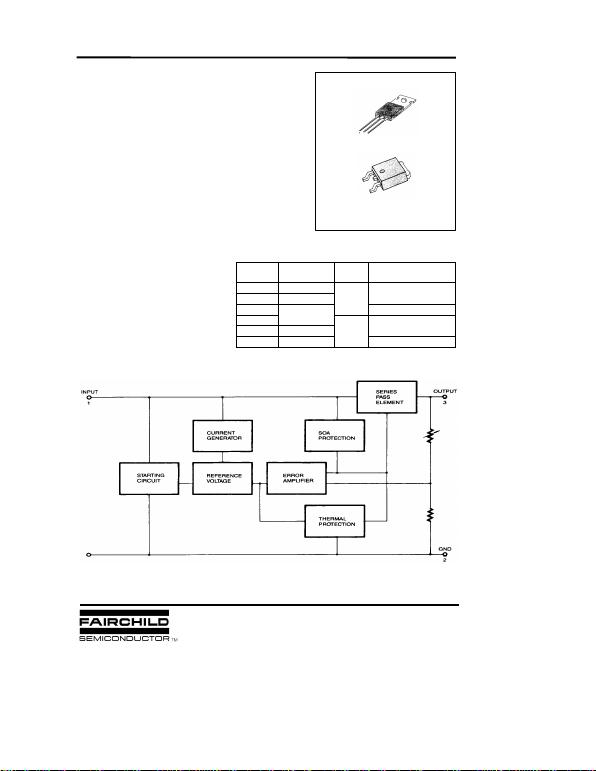LM78XX (KA78XX, MC78XX) FIXED VOLTAGE REGULATOR (POSITIVE)
3-TERMINAL 1A POSITIVE
VOLTAGE REGULATORS
The LM78XX series of three-terminal positive regulators are available in
the TO-220/D-PAK package and with several fixed output voltages, making
them useful in a wide range of applications. Each type employs internal
current limiting, thermal shut-down and safe area protection, making it
essentially indestructible. If adequate heat sinking is provided, they can
deliver over 1A output current. Although designed primarily as fixed voltage
regulators, these devices can be used with external components to obtain
FEATURES
Output Current up to 1A
Output Voltages of 5, 6, 8, 9, 10, 11, 12, 15, 18, 24V
Short Circuit Protection
Output Transistor SOA Protection
KA78XXCT ± 4%
KA78XXAT± 2%
KA78XXIT
TO-220
-40 ~ +125 °C
KA78XXR
KA78XXAR± 2%
KA78XXIR± 4%
D-PAK
-40 ~ +125 °C
0 ~ +125
°
C
0 ~ +125
°
C
TO-220
D-PAK
1: Input 2: GND 3: Output
BLOCK DIAGRAM
1
ORDERING INFORMATION
Device
Operating Temperature
±
4%
Output Voltage
Tolerance
Packag
e
1999 Fairchild Semiconductor Corporation
Rev. B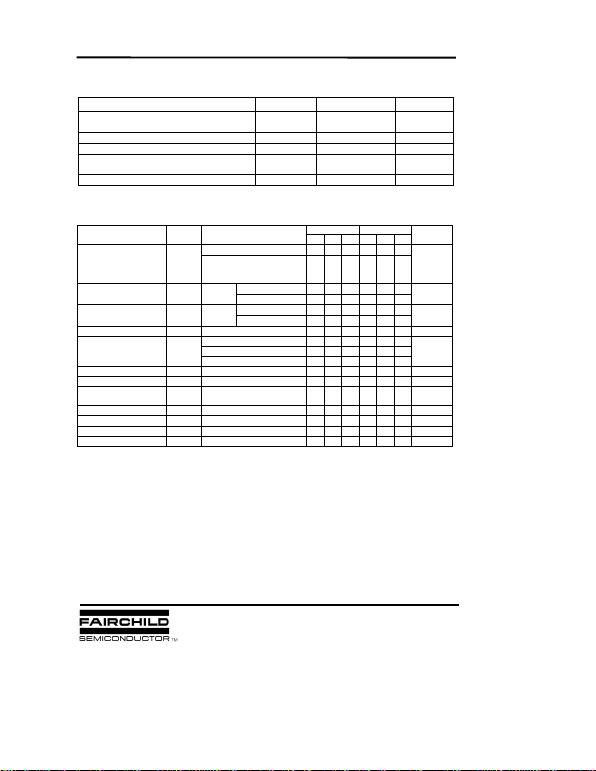LM78XX (KA78XX, MC78XX) FIXED VOLTAGE REGULATOR (POSITIVE)
ABSOLUTE MAXIMUM RATINGS (TA = +25°C, unless otherwise specified)
LM7805/I/R/RI ELECTRICAL CHARACTERISTICS
(Refer to test circuit, TMIN < TJ < TMAX, IO = 500mA, VI = 10V, CI= 0.33µF, CO= 0.1µF, unless otherwise specified)
* TMIN <TJ <TMAX
LM78XXI/RI: TMIN= - 40 °C, TMAX = +125 °C
LM78XX/R: TMIN= 0 °C, TMAX= +125 °C
* Load and line regulation are specified at constant junction temperature. Changes in VO due to heating effects
must be taken into account separately. Pulse testing with low duty is used.
Characteristic Symbol Value Unit
Input Voltage (for VO = 5V to 18V)
(for VO = 24V) VI
VI
35
40 V
V
Thermal Resistance Junction-Cases RθJC 5°C/W
Thermal Resistance Junction-Air RθJA 65 °C/W
Operating Temperature Range KA78XX/A/R/RA
KA78XXI/RI TOPR 0 ~ +125
-40 ~ +125 °C
°C
Storage Temperature Range TSTG -65 ~ +150 °C
LM7805I LM7805
Min Typ Max Min Typ Max
TJ =+25 °C4.8 5.0 5.2 4.8 5.0 5.2
Output Voltage VO5.0mA IO 1.0A, PO 15W
VI = 7V to 20V
VI = 8V to 20V 4.75 5.0 5.25 4.75 5.0 5.25 V
VO = 7V to 25V 4.0 100 4.0 100
VI = 8V to 12V 1.6 50 1.6 50
IO = 5.0mA to1.5A 9 100 9 100
IO =250mA to 750mA 4 50 4 50
Quiescent Current IQTJ =+25 °C5.0 85.0 8mA
IO = 5mA to 1.0A 0.03 0.5 0.03 0.5
Quiescent Current Change IQVI= 7V to 25V 0.3 1.3 mA
VI= 8V to 25V 0.3 1.3
Output Voltage Drift VO/TIO= 5mA -0.8 -0.8 mV/ °C
Output Noise Voltage VNf = 10Hz to 100Khz, TA=+25 °C42 42 µV/Vo
Ripple
Rejection RR f = 120Hz
VO = 8 to 18V 62 73 62 73 dB
Dropout Voltage VOIO = 1A, TJ =+25 °C2 2 V
Output Resistance ROf = 1KHz 15 15 m
Short Circuit Current ISC VI = 35V, TA =+25 °C230 230 mA
Peak Current IPK TJ =+25 °C2.2 2.2 A
Characteristic Symbol Test Conditions Unit
Line Regulation
VO
VO
TJ=+25
°
C
TJ=+25
°
C
mV
mV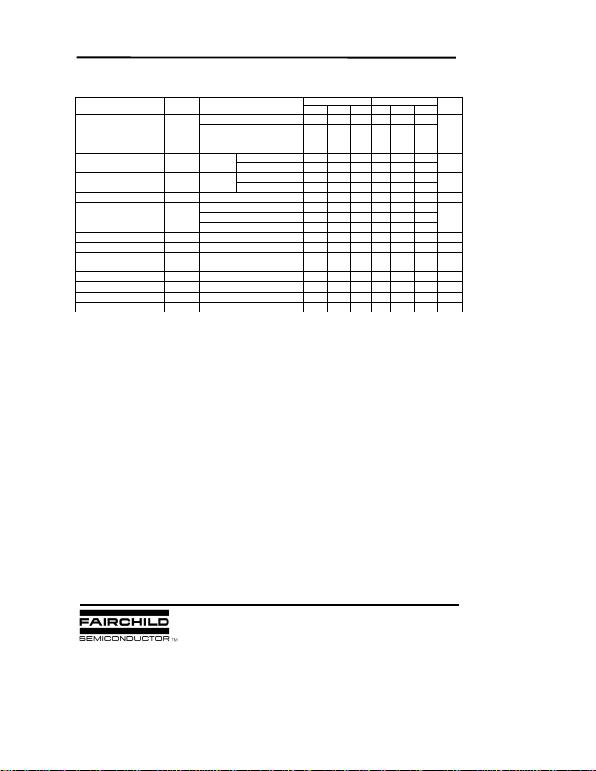LM78XX (KA78XX, MC78XX) FIXED VOLTAGE REGULATOR (POSITIVE)
LM7806/I/R/RI ELECTRICAL CHARACTERISTICS
(Refer to test circuit, TMIN <TJ <TMAX, IO=500mA, VI= 11V CI= 0.33µF, CO= 0.1µF, unless otherwise specified)
* TMIN <TJ <TMAX
LM78XXI/RI: TMIN= - 40 °C, TMAX = +125 °C
LM78XX/R: TMIN= 0 °C, TMAX= +125 °C
* Load and line regulation are specified at constant, junction temperature. Change in VO due to heating effects must be taken
into account separately. Pulse testing with low duty is used.
LM7806I LM7806
Min Typ Max Min Typ Max
TJ =+25 °C5.75 6.0 6.25 5.75 6.0 6.25
Output Voltage VO5.0mA IO 1.0A, PD 15W
VI = 8.0V to 21V
VI = 9.0V to 21V 5.7 6.0 6.3 5.7 6.0 6.3 V
VI = 8V to 25V 5 120 5 120
VI = 9V to 13V 1.5 60 1.5 60
IO =5mA to 1.5A 9 120 9 120
IO =250mA to750A 3 60 3 60
Quiescent Current IQTJ =+25 °C5.0 85.0 8mA
IO = 5mA to 1A 0.5 0.5
Quiescent Current Change IQVI = 8V to 25V 1.3 mA
VI = 9V to 25V 1.3
Output Voltage Drift VO/TIO = 5mA -0.8 -0.8 mV/ °C
Output Noise Voltage VNf = 10Hz to 100Khz, TA =+25 °C45 45 µV/V
O
Ripple
Rejection RR f = 120Hz
VI = 9V to 19V 59 75 59 75 dB
Dropout Voltage VDIO = 1A, TJ =+25 °C2 2 V
Output Resistance RDf = 1KHz 19 19 m
Short Circuit Current ISC VI= 35V, TA=+25°C250 250 mA
Peak Current IPK T
J
=+25 °C2.2 2.2 A
Line Regulation
VO
VO
TJ=+25
°
C
TJ=+25
°
C
mV
mV
Characteristic Symbol Test Conditions Unit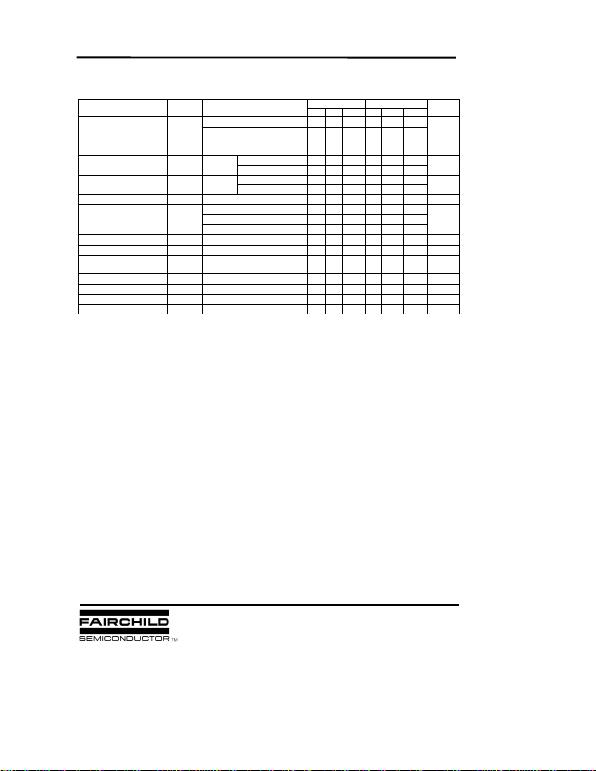LM78XX (KA78XX, MC78XX) FIXED VOLTAGE REGULATOR (POSITIVE)
LM7808/I/R/RI ELECTRICAL CHARACTERISTICS
(Refer to test Circuit, TMIN <TJ< TMAX, IO = 500mA, VI = 14V, CI = 0.33µF, CO= 0.1µF, unless otherwise specified)
* TMIN <TJ <TMAX
LM78XXI/RI: TMIN= - 40 °C, TMAX = +125 °C
LM78XX/R: TMIN= 0 °C, TMAX= +125 °C
* Load and line regulation are specified at constant, junction temperature. Change in VO due to heating effects must be taken
into account separately. Pulse testing with low duty is used.
LM7808I LM7808
Min Typ Max Min Typ Max
TJ =+25 °C7.7 8.0 8.3 7.7 8.0 8.3
Output Voltage VO5.0mA IO 1.0A, PO 15W
VI = 10.5V to 23V
VI = 11.5V to 23V 7.6 8.0 8.4 7.6 8.0 8.4 V
VI = 10.5V to 25V 5.0 160 5.0 160
VI = 11.5V to 17V 2.0 80 2.0 80
IO = 5.0mA to 1.5A 10 160 10 160
IO= 250mA to 750mA 5.0 80 5.0 80
Quiescent Current IQTJ =+25 °C5.0 85.0 8mA
IO = 5mA to 1.0A 0.05 0.5 0.05 0.5
Quiescent Current Change IQVI = 10.5A to 25V 0.5 1.0 mA
VI = 11.5V to 25V 0.5 1.0
Output Voltage Drift VO/TIO = 5mA -0.8 -0.8 mV/ °C
Output Noise Voltage VNf = 10Hz to 100Khz, TA =+25 °C52 52 µV/Vo
Ripple
Rejection RR f = 120Hz, VI= 11.5V to 21.5 56 73 56 73 dB
Dropout Voltage VDIO = 1A, TJ=+25 °C2 2 V
Output Resistance ROf = 1KHz 17 17 m
Short Circuit Current ISC VI= 35V, TA =+25 °C230 230 mA
Peak Current IPK T
J
=+25 °C2.2 2.2 A
Characteristic Symbol Test Conditions Unit
Line Regulation
VO
VO
TJ =+ 25
°
C
TJ = +25
°
C
mV
mV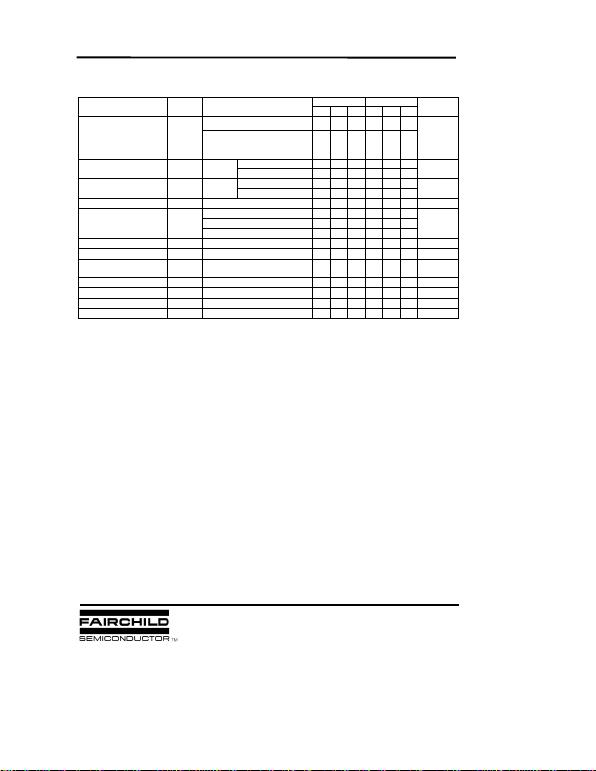LM78XX (KA78XX, MC78XX) FIXED VOLTAGE REGULATOR (POSITIVE)
LM7809/I/R/RI ELECTRICAL CHARACTERISTICS
(Refer to test circuit. TMIN < TJ <TMAX, IO= 500mA, VI= 15V, CI = 0.33µF, CO = 0.1µF. unless otherwise specified)
* TMIN <TJ <TMAX
LM78XXI/RI: TMIN= - 40 °C, TMAX = +125 °C
LM78XX/R: TMIN= 0 °C, TMAX= +125 °C
* Load and line regulation are specified at constant, junction temperature. Change in VO due to heating effects must be taken
into account separately. Pulse testing with low duty is used.
LM7809I LM7809
Min Typ Max Min Typ Max
TJ =+25 °C8.65 99.35 8.65 99.35
Output Voltage VO5.0mA IO 1.0A, PD 15W
VI= 11.5V to 24V
VI = 12.5V to 24V 8.6 99.4 8.6 99.4 V
VI = 11.5V to 25V 6 180 6 180
VI = 12V to 25v 2 90 2 90
IO = 5mA to 1.5A 12 180 12 180
IO = 250mA to 750mA 4 90 4 90
Quiescent Current IQTJ=+25 °C5.0 85.0 8mA
IO = 5mA to 1.0A 0.5 0.5
Quiescent Current Change IQVI = 11.5V to 26V 1.3 mA
VI = 12.5V to 26V 1.3
Output Voltage Drift VO/TIO = 5mA -1 -1 mV/ °C
Output Noise Voltage VNf = 10Hz to 100Khz, TA =+25 °C58 58 µV/VO
Ripple
Rejection RR f = 120Hz
VI = 13V to 23V 56 71 56 71 dB
Dropout Voltage VDIO = 1A, TJ=+25 °C2 2 V
Output Resistance ROf = 1KHz 17 17 m
Short Circuit Current ISC VI= 35V, TA =+25 °C250 250 mA
Peak Current IPK TJ= +25 °C2.2 2.2 A
Characteristic Symbol Test Conditions
Line Regulation
VO
VO
TJ=+25
°
C
TJ=+25
°
C
mV
mV
Unit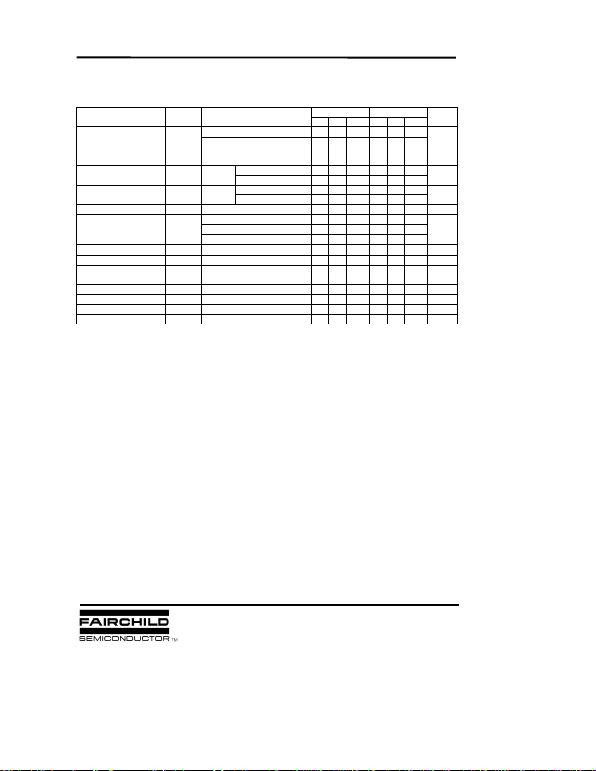LM78XX (KA78XX, MC78XX) FIXED VOLTAGE REGULATOR (POSITIVE)
LM7810/I/R/RI ELECTRICAL CHARACTERISTICS
(Refer to test circuit, TMIN <TJ <TMAX, IO= 500mA, VI =16V, CI = 0.33µF, CO= 0.1µF, unless otherwise specified)
* TMIN <TJ <TMAX
LM78XXI/RI: TMIN= - 40 °C, TMAX = +125 °C
LM78XX/R: TMIN= 0 °C, TMAX= +125 °C
* Load and line regulation are specified at constant, junction temperature. Change in VO due to heating effects must be taken
into account separately. Pulse testing with low duty is used.
LM7810I LM7810
Min Typ Max Min Typ Max
TJ =+25 °C9.6 10 10.4 9.6 10 10.4
Output Voltage VO5.0mA IO1.0A, PD 15W
VI = 12.5V to 25V
VI= 13.5V to 25V 9.5 10 10.5 9.5 10 10.5 V
VI = 12.5V to 25V 10 200 10 200
VI = 13V to 25V 3 100 3 100
IO = 5mA to 1.5A 12 200 12 200
IO = 250mA to 750mA 4 400 4 400
Quiescent Current IQTJ =+25 °C5.1 85.1 8mA
IO = 5mA to 1.0A 0.5 0.5
Quiescent Current Change IQVI = 12.5V to 29V 1.0 mA
VI = 13.5V to 29V 1.0
Output Voltage Drift VO/TIO = 5mA -1 -1 mV/ °C
Output Noise Voltage VNf = 10Hz to 100Khz, TA =+25 °C58 58 µV/Vo
Ripple
Rejection RR f = 120Hz
VI = 13V to 23V 56 71 56 71 dB
Dropout Voltage VDIO = 1A, TJ=+25 °C2 2 V
Output Resistance ROf = 1KHz 17 17 m
Short Circuit Current ISC VI = 35V, TA=+25 °C250 250 mA
Peak Current IPK T
J
=+25 °C2.2 2.2 A
Unit
Line Regulation
VO
VO
TJ =+25
°
C
TJ =+25
°
C
Characteristic Symbol Test Conditions
mV
mV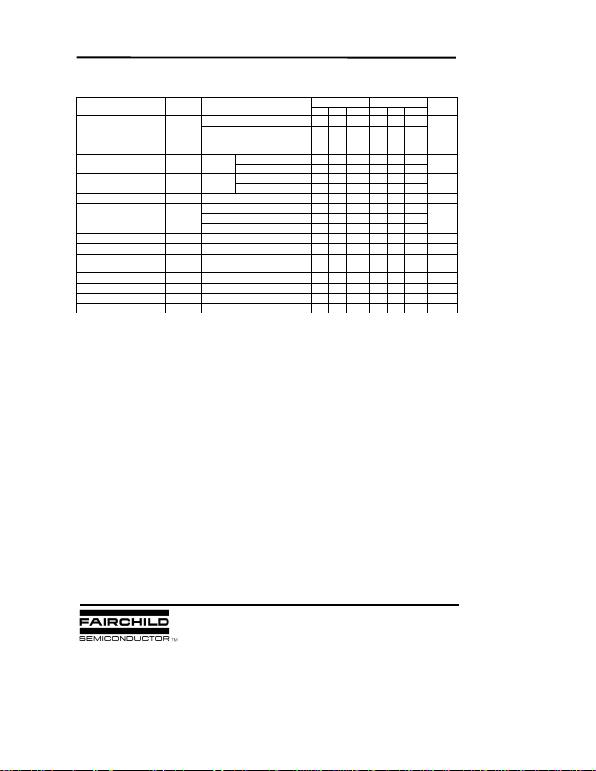LM78XX (KA78XX, MC78XX) FIXED VOLTAGE REGULATOR (POSITIVE)
LM7811/I/R/RI ELECTRICAL CHARACTERISTICS
(Refer to test circuit, TMIN<TJ<TMAX, IO = 500mA, VI=18V, CI=0.33µF, CO = 0.IµF, unless otherwise specified)
* TMIN <TJ <TMAX
LM78XXI/RI: TMIN= - 40 °C, TMAX = +125 °C
LM78XX/R: TMIN= 0 °C, TMAX= +125 °C
* Load and line regulation are specified at constant, junction temperature. Change in VO due to heating effects must be taken
into account separately. Pulse testing with low duty is used.
LM7811I LM7811
Min Typ Max Min Typ Max
TJ =+25 °C10.6 11 11.4 10.6 11 11.4
Output Voltage VO5.0mA IO 1.0A, PD 15W
VI = 13.5V to 26V
VI= 14.5V to 26V 10.5 11 11.5 10.5 11 11.5 V
VI = 13.5V to 25V 10 220 10 220
VI = 14V to 21V 3.0 110 3 110
IO = 5.0mA to 1.5A 12 220 12 220
IO = 250mA to 750mA 4 110 4 110
Quiescent Current IQTJ =+25 °C5.1 85.1 8mA
IO = 5mA to 1.0A 0.5 0.5
Quiescent Current Change IQVI = 13.5V to 29V 1.0 mA
VI = 14.5V to 29V 1.0
Output Voltage Drift VO/TIO = 5mA -1 -1 mV/ °C
Output Noise Voltage VNf = 10Hz to 100Khz, TA =+25 °C70 70 µV/VO
Ripple
Rejection RR f = 120Hz
VI = 14V to 24V 55 71 55 71 dB
Dropout Voltage VDIO = 1A, TJ=+25 °C2 2 V
Output Resistance ROf = 1KHz 18 18 m
Short Circuit Current ISC VI = 35V, TA=+25 °C250 250 mA
Peak Current IPK T
J
=+25 °C2.2 2.2 A
Unit
Line Regulation
VO
VO
TJ =+25
°
C
TJ =+25
°
C
Characteristic Symbol Test Conditions
mV
mVLM78XX (KA78XX, MC78XX) FIXED VOLTAGE REGULATOR (POSITIVE)
LM7812/I/R/RI ELECTRICAL CHARACTERISTICS
(Refer to test circuit, TMIN <TJ <TMAX, IO=500mA, VI=19V, CI= 0.33µF, CO= 0.1.µF, unless otherwise specified)
TMIN <TJ <TMAX
LM78XXI/RI: TMIN= - 40 °C, TMAX = +125 °C
LM78XX/R: TMIN= 0 °C, TMAX= +125 °C
* Load and line regulation are specified at constant, junction temperature. Change in VO due to heating effects must be taken
into account separately. Pulse testing with low duty is used.
LM7812I LM7812
Min Typ Max Min Typ Max
TJ =+25 °C11.5 12 12.5 11.5 12 12.5
Output Voltage VO5.0mA IO1.0A, PD15W
VI = 14.5V to 27V
VI= 15.5V to 27V 11.4 12 12.6 11.4 12 12.6 V
VI = 14.5V to 30V 10 240 10 240
VI = 16V to 22V 3.0 120 3.0 120
IO = 5mA to 1.5A 11 240 11 240
IO = 250mA to 750mA 5.0 120 5.0 120
Quiescent Current IQTJ =+25 °C5.1 85.1 8mA
IO = 5mA to 1.0A 0.1 0.5 0.1 0.5
Quiescent Current Change IQVI = 14.5V to 30V 0.5 1.0 mA
VI = 15V to 30V 1.0
Output Voltage Drift VO/TIO = 5mA 0.5 -1 -1 mV/ °C
Output Noise Voltage VNf = 10Hz to 100Khz, TA =+25 °C76 76 mV/VO
Ripple
Rejection RR f = 120Hz
VI = 15V to 25V 55 71 55 71 dB
Dropout Voltage VDIO = 1A, TJ=+25 °C2 2 V
Output Resistance ROf = 1KHz 18 18 m
Short Circuit Current ISC VI = 35V, TA=+25 °C230 230 mA
Peak Current IPK T
J
= +25 °C2.2 2.2 A
Unit
Line Regulation
VO
VO
TJ =+25
°
C
TJ =+25
°
C
Characteristic Symbol Test Conditions
mV
mV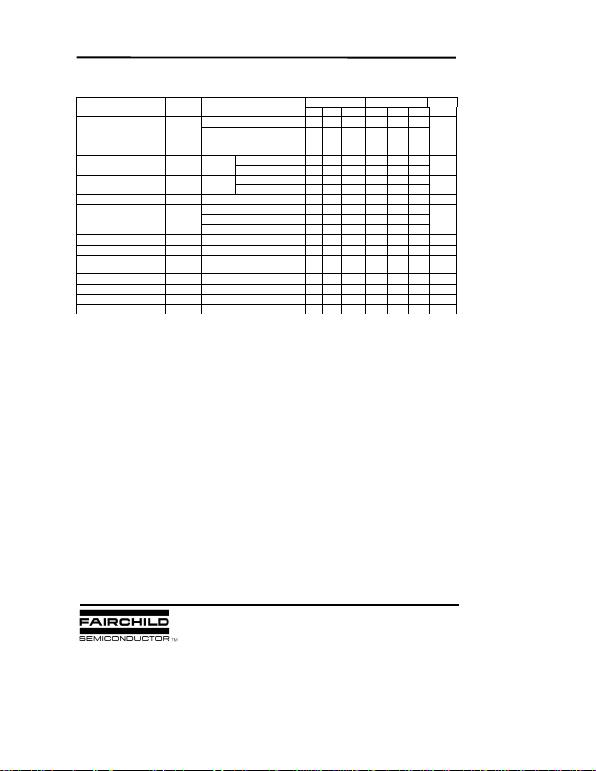LM78XX (KA78XX, MC78XX) FIXED VOLTAGE REGULATOR (POSITIVE)
LM7815/I/R/RI ELECTRICAL CHARACTERISTICS
(Refer to test circuit, TMIN<TJ<TMAX, IO =500mA, VI =23V, CI =0.33µF, CO =0.1µF, unless otherwise specified)
* TMIN <TJ <TMAX
LM78XXI/RI: TMIN= - 40 °C, TMAX = +125 °C
LM78XX/R: TMIN= 0 °C, TMAX= +125 °C
* Load and line regulation are specified at constant, junction temperature. Change in VO due to heating effects must be taken
into account separately. Pulse testing with low duty is used.
LM7815I LM7815
Min Typ Max Min Typ Max
TJ =+25 °C14.4 15 15.6 14.4 15 15.6
Output Voltage VO5.0mA IO1.0A, PD15W
VI = 17.5V to 30V
VI= 18.5V to 30V 14.2
515 15.75 14.25 15 15.75 V
VI = 17.5V to 30V 11 300 11 300
VI = 20V to 26V 3 150 3 150
IO = 5mA to 1.5A 12 300 12 300
I
O
= 250mA to 750mA 4 150 4 150
Quiescent Current IQTJ =+25 °C5.2 85.2 8mA
IO = 5mA to 1.0A 0.5 0.5
Quiescent Current Change IQVI = 17.5V to 30V 1.0 mA
VI = 18.5V to 30V 1.0
Output Voltage Drift VO/TIO = 5mA -1 -1 mV/ °C
Output Noise Voltage VNf = 10Hz to 100Khz, TA =+25 °C90 90 µV/VO
Ripple
Rejection RR f = 120Hz
VI = 18.5V to 28.5V 54 70 54 70 dB
Dropout Voltage VDIO = 1A, TJ=+25 °C2 2 V
Output Resistance ROf = 1KHz 19 19 m
Short Circuit Current ISC VI = 35V, TA=+25 °C250 250 mA
Peak Current IPK T
J
=+25 °C2.2 2.2 A
Unit
Line Regulation
VO
VO
TJ =+25
°
C
TJ =+25
°
C
Characteristic Symbol Test Conditions
mV
mV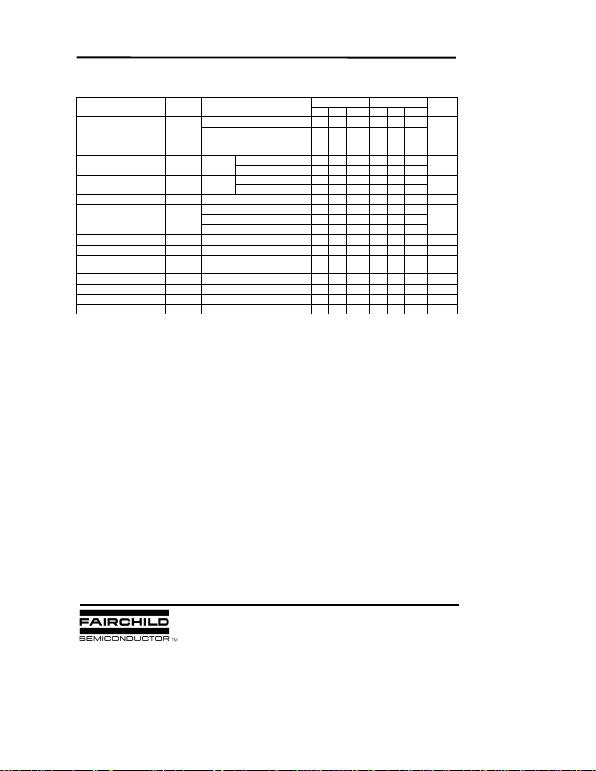LM78XX (KA78XX, MC78XX) FIXED VOLTAGE REGULATOR (POSITIVE)
LM7818/I/R/RI ELECTRICAL CHARACTERISTICS
(Refer to test circuit, TMIN<TJ<TMAX, IO =500mA, VI =27V, CI =0.33µF, CO =0.1µF, unless otherwise specified)
* TMIN <TJ <TMAX
LM78XXI/RI: TMIN= - 40 °C, TMAX = +125 °C
LM78XX/R: TMIN= 0 °C, TMAX= +125 °C
* Load and line regulation are specified at constant, junction temperature. Change in VO due to heating effects must be taken
into account separately. Pulse testing with low duty is used.
LM7818I LM7818
Min Typ Max Min Typ Max
TJ =+25 °C17.3 18 18.7 17.3 18 18.7
Output Voltage VO5.0mA IO 1.0A, PD 15W
VI = 21V to 33V
VI= 22V to 33V 17.1 18 18.9 17.1 18 18.9 V
VI = 21V to 33V 15 360 15 360
VI = 24V to 30V 5 180 5 180
IO = 5mA to 1.5A 15 360 15 360
IO = 250mA to 750mA 5.0 180 5.0 180
Quiescent Current IQTJ =+25 °C5.2 85.2 8mA
IO = 5mA to 1.0A 0.5 0.5
Quiescent Current Change IQVI = 21V to 33V 1mA
VI = 22V to 33V 1.0
Output Voltage Drift VO/TIO = 5mA -1 -1 mV/ °C
Output Noise Voltage VNf = 10Hz to 100Khz, TA =+25 °C110 110 µV/VO
Ripple
Rejection RR f = 120Hz
VI = 22V to 32V 53 69 53 69 dB
Dropout Voltage VDIO = 1A, TJ=+25 °C2 2 V
Output Resistance ROf = 1KHz 22 22 m
Short Circuit Current ISC VI = 35V, TA=+25 °C250 250 mA
Peak Current IPK T
J
=+25 °C2.2 2.2 A
Unit
Line Regulation
VO
VO
TJ =+25
°
C
TJ =+25
°
C
Characteristic Symbol Test Conditions
mV
mVLM78XX (KA78XX, MC78XX) FIXED VOLTAGE REGULATOR (POSITIVE)
LM7824/I/R/RI ELECTRICAL CHARACTERISTICS
(Refer to test circuit, TMIN<TJ<TMAX, IO = 500mA, VI = 33V, CI = 0.33µF, CO = 0.1µF, unless otherwise specified)
* TMIN <TJ <TMAX
LM78XXI/RI: TMIN= - 40 °C, TMAX = +125 °C
LM78XX/R: TMIN= 0 °C, TMAX= +125 °C
* Load and line regulation are specified at constant, junction temperature. Change in VO due to heating effects must be taken
into account separately. Pulse testing with low duty is used.
LM7824I LM7824
Min Typ Max Min Typ Max
TJ =+25 °C23 24 25 23 24 25
Output Voltage VO5.0mA IO 1.0A, PD 15W
VI = 27V to 38V
VI= 28V to 38V 22.8 24 25.2 22.8 24 25.25 V
VI = 27V to 38V 17 480 17 480
VI = 30V to 36V 6 240 6 240
IO = 5mA to 1.5A 15 480 15 480
IO = 250mA to 750mA 5.0 240 5.0 240
Quiescent Current IQTJ =+25 °C5.2 85.2 8mA
IO = 5mA to 1.0A 0.1 0.5 0.1 0.5
Quiescent Current Change IQVI = 27V to 38V 0.5 1mA
VI = 28V to 38V 0.5 1
Output Voltage Drift VO/TIO = 5mA -1.5 -1.5 mV/ °C
Output Noise Voltage VNf = 10Hz to 100KHz, TA =+25 °C160 60 µV/VO
Ripple
Rejection RR f = 120Hz
VI = 28V to 38V 50 67 50 67 dB
Dropout Voltage VDIO = 1A, TJ=+25 °C2 2 V
Output Resistance ROf = 1KHz 28 28 m
Short Circuit Current ISC VI = 35V, TA=+25 °C230 230 mA
Peak Current IPK T
J
=+25 °C2.2 2.2 A
Unit
Line Regulation
VO
VO
TJ =+25
°
C
TJ =+25
°
C
Characteristic Symbol Test Conditions
mV
mV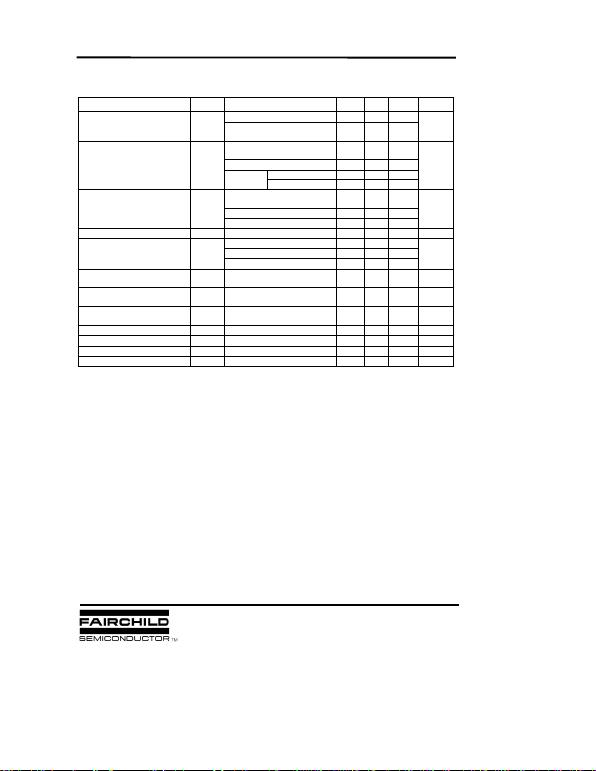LM78XX (KA78XX, MC78XX) FIXED VOLTAGE REGULATOR (POSITIVE)
LM7805A/RA ELECTRICAL CHARACTERISTICS
(Refer to the test circuits. TJ = 0 to +I25 °C, IO = 1A, V I = 10V, C I= 0.33µF, C O= 0.1µF, unless otherwise specified)
*Load and line regulation are specified at constant, junction temperature. Change in VO due to heating effects must be taken
into account separately. Pulse testing with low duty is used.
Characteristic Symbol Test Conditions Min Typ Max Unit
TJ =+25 °C4.9 55.1
Output Voltage VO IO = 5mA to 1A, PD 5W
VI = 7.5 to 20V 4.8 55.2 V
VI = 7.5 to 25V
IO = 500mA 5 50
Line Regulation VO VI = 8V to 12V 3 50 V
VI= 7.3V to 25V 5 50
VI= 8V to 12V 1.5 25
TJ =+25 °C
IO = 5mA to 1.5A 9 100
IO = 5mA to 1A 9 100
IO = 250 to 750mA 4 50
Quiescent Current IQ TJ =+25 °C5.0 6mA
IO = 5mA to 1A 0.5
Quiescent Current Change IQ VI = 8 V to 25V, IO = 500mA 0.8 mA
VI = 7.5V to 20V, TJ =+25 °C0.8
Output Voltage Drift -0.8 mV/ °C
Output Noise Voltage VN f = 10Hz to 100KHz
TA =+25 °C10
Ripple Rejection RR f = 120Hz, IO = 500mA
VI = 8V to 18V 68 dB
Dropout Voltage VD IO = 1A, TJ =+25 °C2V
Output Resistance RO f = 1KHz 17 m
Short Circuit Current ISC VI= 35V, TA =+25 °C250 mA
Peak Current IPK TJ= +25 °C2.2 A
VO
TJ =+25
°
C
V/
TIO = 5mA
µ
V/VO
V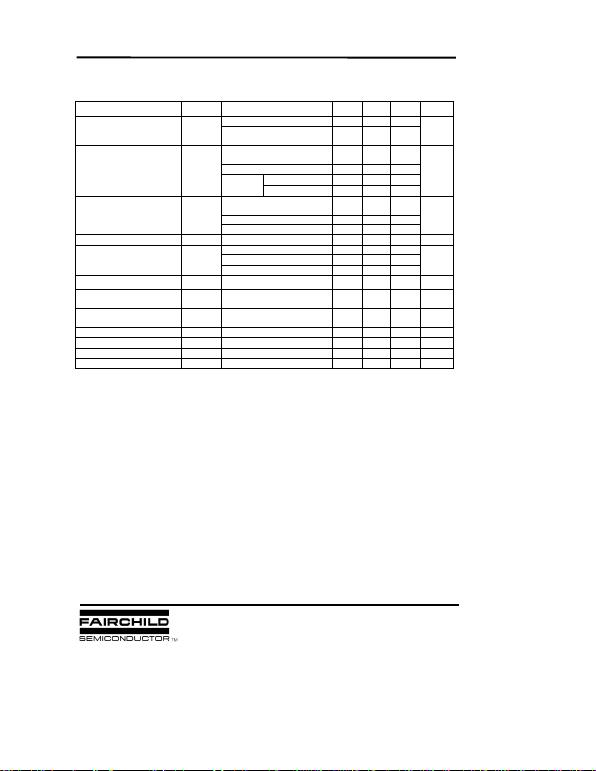LM78XX (KA78XX, MC78XX) FIXED VOLTAGE REGULATOR (POSITIVE)
LM7806A/RA ELECTRICAL CHARACTERISTICS
(Refer to the test circuits. TJ = 0 to+150 °C, IO = 1A, V I = 11V, C I= 0.33µF, C O= 0.1µF, unless otherwise specified)
* Load and line regulation are specified at constant, junction temperature. Change in VO due to heating effects must be taken
into account separately. Pulse testing with low duty is used.
Characteristic Symbol Test Conditions Min Typ Max Unit
TJ =+25 °C5.58 66.12
Output Voltage VO IO = 5mA to 1A, PD 15W
VI = 8.6 to 21V 5.76 66.24 V
VI= 8.6 to 25V
IO = 500mA 5 60
Line Regulation VO VI= 9V to 13V 3 60 mV
VI= 8.3V to 21V 5 60
VI= 9V to 13V 1.5 30
TJ =+25 °C
IO = 5mA to 1.5A 9 100
IO = 5mA to 1A 4 100
IO = 250 to 750mA 5.0 50
Quiescent Current IQ TJ =+25 °C4.3 6mA
IO = 5mA to 1A 0.5
Quiescent Current Change IQ VI = 9V to 25V, IO = 500mA 0.8 mA
VI= 8.5V to 21V, TJ =+25 °C0.8
Output Voltage Drift IO = 5mA -0.8 mV/ °C
Output Noise Voltage VN f = 10Hz to 100KHz
TA =+25 °C10 µ V/VO
Ripple Rejection RR f = 120Hz, IO = 500mA
VI = 9V to 19V 65 dB
Dropout Voltage VD IO = 1A, TJ =+25 °C2V
Output Resistance RO f = 1KHz 17 m
Short Circuit Current ISC VI= 35V, TA =+25 °C250 mA
Peak Current IPK TJ=+25 °C2.2 A
VO
TJ =+25
°
C
V/
T
mV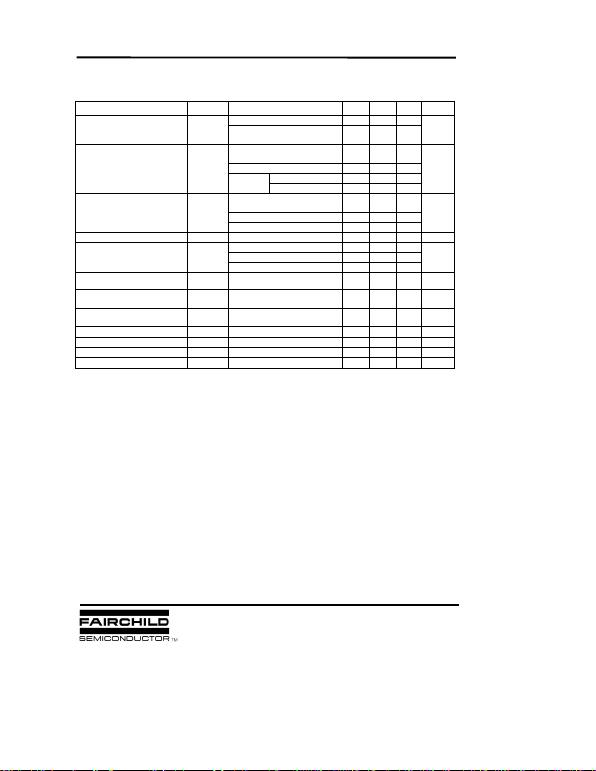LM78XX (KA78XX, MC78XX) FIXED VOLTAGE REGULATOR (POSITIVE)
LM7808A/RA ELECTRICAL CHARACTERISTICS
(Refer to the test circuits. TJ = 0 to+150 °C, IO = 1A, V I = 14V, C I = 0.33µF, C O=0.1µF, unless otherwise specified)
* Load and line regulation are specified at constant, junction temperature. Change in VO due to heating effects must be taken
into account separately. Pulse testing with low duty is used.
Characteristic Symbol Test Conditions Min Typ Max Unit
TJ =+25 °C7.84 88.16
Output Voltage VO IO = 5mA to 1A, PD 15W
VI = 8.6 to 21V 7.7 88.3 V
VI= 10.6 to 25V
IO = 500mA 6 80
Line Regulation VO VI= 11to 17V 3 80 mV
VI= 10.4V to 23V 6 80
VI= 11V to 17V 2 40
TJ =+25 °C
IO = 5mA to 1.5A 12 100
IO = 5mA to 1A 12 100
IO = 250 to 750mA 5 50
Quiescent Current IQ TJ =+25 °C5.0 6mA
IO = 5mA to 1A 0.5
Quiescent Current Change IQ VI = 11V to 25V, IO = 500mA 0.8 mA
VI= 10.6V to 23V, TJ =+25 °C0.8
Output Voltage Drift IO = 5mA -0.8 mV /°C
Output Noise Voltage VN f = 10Hz to 100KHz
TA =+25 °C10 µV/VO
Ripple Rejection RR f = 120Hz, IO = 500mA
VI = 11.5V to 21.5V 62 dB
Dropout Voltage VD IO = 1A, TJ =+25 °C2V
Output Resistance RO f = 1KHz 18 m
Short Circuit Current ISC VI= 35V, TA =+25°C250 mA
Peak Current IPK TJ=+25 °C2.2 A
VO
TJ =+25
°
C
V/
T
mV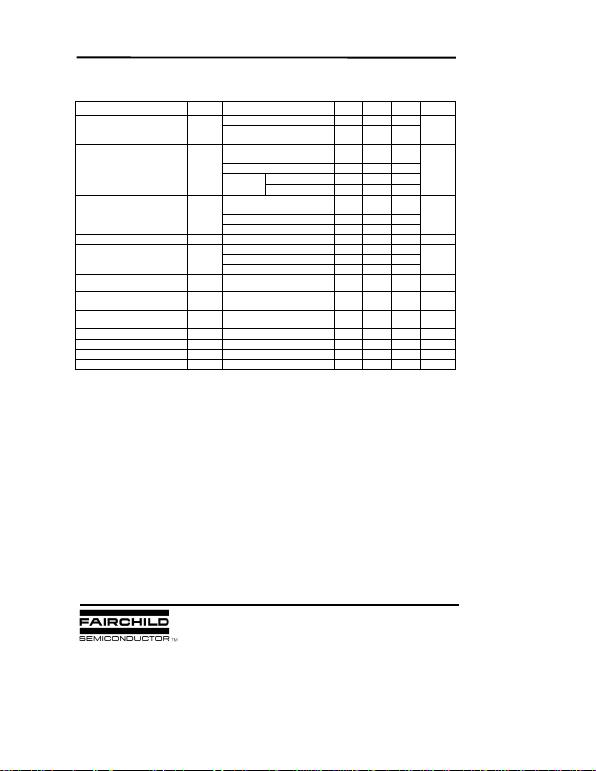LM78XX (KA78XX, MC78XX) FIXED VOLTAGE REGULATOR (POSITIVE)
LM7809A/RA ELECTRICAL CHARACTERISTICS
(Refer to the test circuits. TJ = 0 to +125 °C, IO = 1A, V I = 15V, C I = 0.33µF, C O = 0.1µF, unless otherwise specified)
* Load and line regulation are specified at constant, junction temperature. Change in VO due to heating effects must be taken
into account separately. Pulse testing with low duty is used.
Characteristic Symbol Test Conditions Min Typ Max Unit
TJ =+25 °C8.82 9.0 9.18
Output Voltage VO IO = 5mA to 1A, PD 15W
VI = 11.2 to 24V 8.65 9.0 9.35 V
VI= 11.7 to 25V
IO = 500mA 6 90
Line Regulation VO VI= 12.5 to 19V 4 45 mV
VI= 11.5V to 24V 6 90
VI= 12.5V to 19V 2 45
TJ =+25 °C
IO = 5mA to 1.0A 12 100
IO = 5mA to 1.0A 12 100
IO = 250 to 750mA 5 50
Quiescent Current IQ TJ =+25 °C5.0 6.0 mA
VI = 11.7V to 25V, TJ=+25 °C0.8
Quiescent Current Change IQVI = 12V to 25V, IO = 500mA 0.8 mA
IO = 5mA to 1.0A 0.5
Output Voltage Drift IO = 5mA -1.0 mV/ °C
Output Noise Voltage VN f = 10Hz to 100KHz
TA =+25 °C10
Ripple Rejection RR f = 120Hz, IO = 500mA
VI = 12V to 22V 62 dB
Dropout Voltage VD IO = 1A, TJ =+25 °C2.0 V
Output Resistance RO f = 1KHz 17 m
Short Circuit Current ISC VI= 35V, TA =+25 °C250 mA
Peak Current IPK TJ=+25 °C2.2 A
VO
TJ =+25
°
C
V/
T
µ
V/VO
mV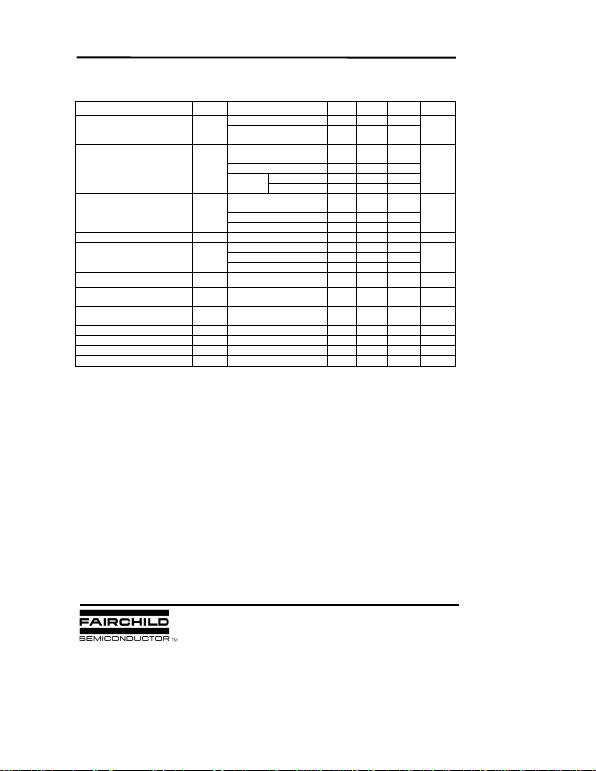LM78XX (KA78XX, MC78XX) FIXED VOLTAGE REGULATOR (POSITIVE)
LM7810A/RA ELECTRICAL CHARACTERISTICS
(Refer to the test circuits. TJ = 0 to+125 °C, IO = 1A, V I = 16V, C I = 0.33µF, CO = 0.1µF, unless otherwise specified)
* Load and line regulation are specified at constant, junction temperature. Change in VO due to heating effects must be taken
into account separately. Pulse testing with low duty is used.
Characteristic Symbol Test Conditions Min Typ Max Unit
TJ =+25 °C9.8 10 10.2
Output Voltage VO IO = 5mA to 1A, PD 15W
VI =12.8 to 25V 9.6 10 10.4 V
VI= 12.8 to 26V
IO = 500mA 8 100
Line Regulation VO VI= 13to 20V 4 50 mV
VI= 12.5V to 25V 8 100
VI= 13V to 20V 3 50
TJ =+25 °C
IO = 5mA to 1.5A 12 100
IO = 5mA to 1.0A 12 100
IO = 250 to 750mA 5 50
Quiescent Current IQ TJ =+25 °C5.0 6.0 mA
VI = 13V to 26V, TJ=+25 °C0.5
Quiescent Current Change IQ VI = 12.8V to 25V, IO = 500mA 0.8 mA
IO = 5mA to 1.0A 0.5
Output Voltage Drift IO = 5mA -1.0 mV °C
Output Noise Voltage VN f = 10Hz to 100KHz
TA =+25 °C10
Ripple Rejection RR f = 120Hz, IO = 500mA
VI = 14V to 24V 62 dB
Dropout Voltage VD IO = 1A, TJ =+25 °C2.0 V
Output Resistance RO f = 1KHz 17 m
Short Circuit Current ISC VI= 35V, TA =+25 °C250 mA
Peak Current IPK TJ=+25 °C2.2 A
VO
TJ =+25
°
C
V/
T
µ
V/VO
mV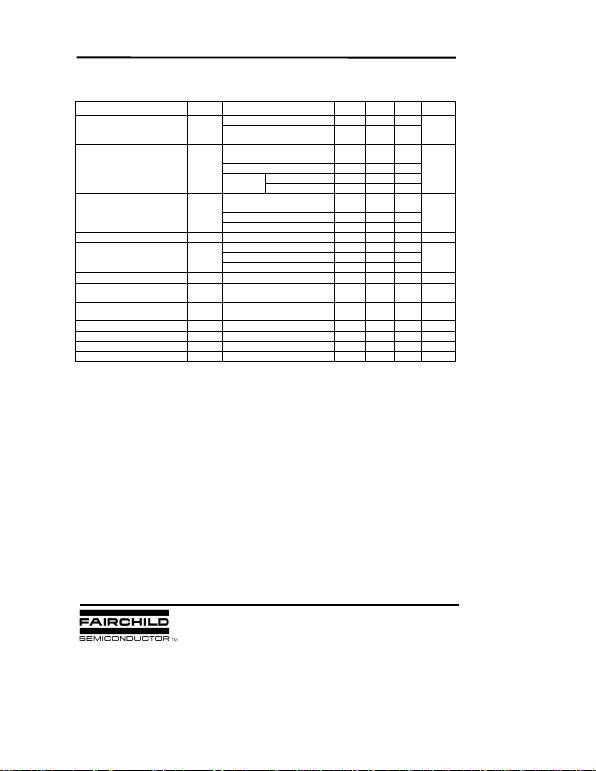LM78XX (KA78XX, MC78XX) FIXED VOLTAGE REGULATOR (POSITIVE)
LM7811A/RA ELECTRICAL CHARACTERISTICS
(Refer to the test circuits. TJ = 0 to +125 °C, IO = 1A, V I = 18V, C I = 0.33µF, C O = 0.1µF, unless otherwise specified)
* Load and line regulation are specified at constant, junction temperature. Change in VO due to heating effects must be taken
into account separately. Pulse testing with low duty is used.
Characteristic Symbol Test Conditions Min Typ Max Unit
TJ =+25 °C10.8 11.0 11.2
Output Voltage VO IO = 5mA to 1A, PD 15W
VI = 13.8 to 26V 10.6 11.0 11.4 V
VI= 12.8 to 26V
IO = 500mA 10 110
Line Regulation VO VI= 15 to 21V 4 55 mV
VI= 13.5V to 26V 10 110
VI= 15V to 21V 3 55
TJ =+25 °C
IO = 5mA to 1.5A 12 100
IO = 5mA to 1.0A 12 100
IO = 250 to 750mA 5 50
Quiescent Current IQ TJ =+25 °C5.1 6.0 mA
VI = 13.8V to 26V, TJ=+25 °C0.8
Quiescent Current Change IQ VI = 14V to 27V, IO = 500mA 0.8 mA
IO = 5mA to 1.0A 0.5
Output Voltage Drift VO/T IO = 5mA -1.0 mV /°C
Output Noise Voltage VN f = 10Hz to 100KHz
TA =+25 °C10
Ripple Rejection RR f = 120Hz, IO = 500mA
VI = 14V to 24V 61 dB
Dropout Voltage VD IO = 1A, TJ =+25 °C2.0 V
Output Resistance RO f = 1KHz 18 m
Short Circuit Current ISC VI= 35V, TA =+25 °C250 mA
Peak Current IPK TJ=+25 °C2.2 A
VO
TJ =+25
°
C
µ
V/VO
mVLM78XX (KA78XX, MC78XX) FIXED VOLTAGE REGULATOR (POSITIVE)
LM7812A/RA ELECTRICAL CHARACTERISTICS
(Refer to the test circuits. TJ = 0 to +125 °C, IO = 1A, V I = 19V, C I = 0.33µF, C O= 0.1µF, unless otherwise specified)
* Load and line regulation are specified at constant, junction temperature. Change in VO due to heating effects must be taken
into account separately. Pulse testing with low duty is used.
Characteristic Symbol Test Conditions Min Typ Max Unit
TJ =+25 °C11.75 12 12.25
Output Voltage VO IO = 5mA to 1A, PD 15W
VI = 14.8 to 27V 11.5 12 12.5 V
VI= 14.8 to 30V
IO = 500mA 10 120
Line Regulation VO VI= 16 to 22V 4 120 mV
VI= 14.5V to 27V 10 120
VI= 16V to 22V 3 60
TJ =+25°C
IO = 5mA to 1.5A 12 100
IO = 5mA to 1.0A 12 100
IO = 250 to 750mA 5 50
Quiescent Current IQ TJ =+25 °C5.1 6.0 mA
VI = 15V to 30V, TJ=+25 °C0.5
Quiescent Current Change IQ VI = 14V to 27V, IO = 500mA 0.8 mA
IO = 5mA to 1.0A 0.8
Output Voltage Drift VO/T IO = 5mA -1.0 mV/ °C
Output Noise Voltage VN f = 10Hz to 100KHz
TA =+25 °C10
Ripple Rejection RR f = 120Hz, IO = 500mA
VI = 14V to 24V 60 dB
Dropout Voltage VD IO = 1A, TJ =+25 °C2.0 V
Output Resistance RO f = 1KHz 18 m
Short Circuit Current ISC VI= 35V, TA =+25 °C250 mA
Peak Current IPK TJ=+25 °C2.2 A
VOmV
µ
V/VO
TJ =+25
°
C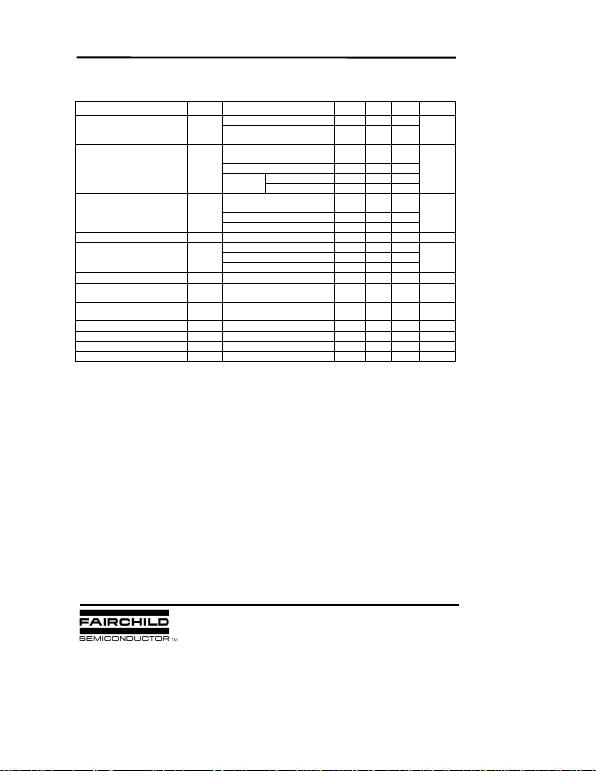LM78XX (KA78XX, MC78XX) FIXED VOLTAGE REGULATOR (POSITIVE)
LM7815A/RA ELECTRICAL CHARACTERISTICS
(Refer to the test circuits. TJ = 0 to +150 °C, IO =1A, V I=23V, C I = 0.33µF, C O=0.1µF, unless otherwise specified)
* Load and line regulation are specified at constant, junction temperature. Change in VO due to heating effects must be taken
into account separately. Pulse testing with low duty is used.
Characteristic Symbol Test Conditions Min Typ Max Unit
TJ =+25 °C14.7 15 15.3
Output Voltage VO IO = 5mA to 1A, PD 15W
VI = 17.7 to 30V 14.4 15 15.6 V
VI= 17.9 to 30V
IO = 500mA 10 150
Line Regulation VO VI= 20 to 26V 5 150 mV
VI= 17.5V to 30V 11 150
VI= 20V to 26V 3 75
TJ =+25 °C
IO = 5mA to 1.5A 12 100
IO = 5mA to 1.0A 12 100
IO = 250 to 750mA 5 50
Quiescent Current IQ TJ =+25 °C5.2 6.0 mA
VI = 17.5V to 30V, TJ =+25 °C0.5
Quiescent Current Change IQ VI = 17.5V to 30V, IO = 500mA 0.8 mA
IO = 5mA to 1.0A 0.8
Output Voltage Drift VO/T IO = 5mA -1.0 mV/ °C
Output Noise Voltage VN f = 10Hz to 100KHz
TA =+25 °C10
Ripple Rejection RR f = 120Hz, IO = 500mA
VI = 18.5V to 28.5V 58 dB
Dropout Voltage VD IO = 1A, TJ =+25 °C2.0 V
Output Resistance RO f = 1KHz 19 m
Short Circuit Current ISC VI= 35V, TA =+25 °C250 mA
Peak Current IPK TJ=+25 °C2.2 A